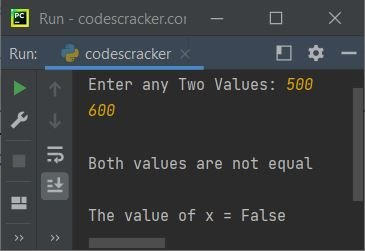# Python False keyword

The "False" keyword in Python is basically a Boolean value, the result of a comparison operation, or the result of any other logical expression whose value evaluates in the form of True or False. For example:

```x = 10
y = 20

print(x==y)
print(x>y)```

The output is:

```False
False```

Note: Python is a case-sensitive language. As a result, keep the first letter of the "False" keyword in capital letters and the remaining letters in small letters.

In logical expressions and conditional statements, the value "False" corresponds to the boolean value False. A variable or function name containing the word "False" is prohibited by Python's naming conventions.

In Python, there are two boolean values: True and False. In order to determine whether or not a given condition holds true, Boolean values are often combined with comparison operators (such as ==, <, >, etc.).

## Python False keyword example

Here is an example of the "False" keyword in Python:

```print("Enter any Two Values: ", end="")
a = input()
b = input()

x = a==b
if x:
print("\nBoth values are equal")
else:
print("\nBoth values are not equal")

print("\nThe value of x =", x)```

The sample run with user input of 500 as the first number and 600 as the second number is shown in the snapshot given below:### Advantages of the "False" keyword in Python

• It can be used in conditional statements to determine whether a condition is true or false.
• It can be used as a default value for function arguments or variables that require a Boolean initialization.
• Utilizing the natural language of Boolean logic can help make code more readable and expressive.

### Disadvantages of the "False" keyword in Python

• Incorrect usage, such as using = instead of == in an equality comparison, can result in errors.
• It can be confusing for novice programmers unfamiliar with Boolean logic or the Python syntax.
• It can be abused or overused, resulting in code that is unnecessarily complex or hard to comprehend.

Python Online Test

« Previous Tutorial Next Tutorial »